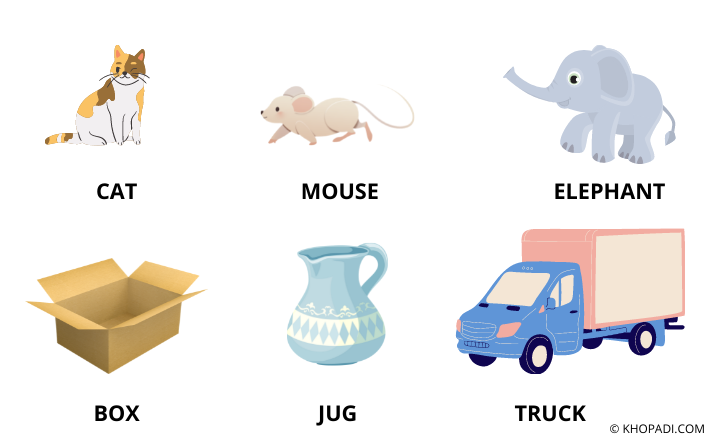# ⚡ DataTypes in MQL

## DataTypes in MQL ⚡

Data is the meaningful information for system development. Data can be of two types majorly either Text Data or Non-Text Data. Non-Text data can be Audio, Video, or Image.

## What is DataType?

DataType is the container type for holding the data. The dataType can be a different type as per the Data Itself.

For Example,

Consider Three Animals:- Cat,  Mouse, Elephant. All three have different sizes.DataType Usage Example MQL

• We can fit "Mouse" in all of the above containers (Box, Jug, Truck).
• We can fit "Cat" in all of the above containers (Box, Truck).
• We can fit "Elephant" in all of the above containers (Truck).
Wait!!

Should I use Truck or Box to fit, Mouse 😂
Yes, if you think about optimization of Space. then you said that we can easily fit our Mouse in Jug. why waste such large space of Box or Truck.

Similarly,

Trading Text Data can be either Numeric [Combination of 0-9 Digits] or String [Combination of A-Z Alphabets.]

## What are the different DataTypes in MQL? [Mostly Used]

Text Data can be further classified by the range of Numbers as Follows:-

No DataType Name Range Storage
1. short From -32,768 to the +32,767 2 bytes (16-Bits)
2. int From -2,147,483,648 to the maximal one is +2,147,483,647 4 bytes (32-Bits)
3. long From -9,223,372,036,854,775,808 to the maximum value is +9,223,372,036,854,775,807 8 bytes (64-Bits)
4. float From 1.175494351e-38 to the maximum value is 3.402823466e+38 4 bytes (32-Bits)
5. double From 2.2250738585072014e-308 to the maximum value is 1.7976931348623158e+308 8 bytes (64-Bits)
6. string - Depends upon the number of Characters used

Let's print some values using the above data types,

### Source Code

``` void OnStart() { // we are printing user-defined variables. short x = 24320 ; long y = 98765432109876543210; double d = 2.3102; string test = "Welcome To Khopadi.com"; Alert(test); Alert("value-x = ", x, "value-y = ", y, "value-d = ", d); } ```

### Explanation of Output

When you try the above source code, then you will find the Alert output mentioned above.
In the example,

• "x" variable is defined as a Short, Printing the value of "x" using Alert Function.
• "y" variable is defined as a Long, Printing the value of "y" using Alert Function.
• "d" variable is defined as a Double, Printing the value of "d" using Alert Function.
• Single line String or "Test" data is also stored in the variable named "test" and Printing its value using Alert Function.
• If you also want to see an example of Integer then you can the example of Addition which is discussed in the previous MQL Forex Programming Tutorial.
If we take an example,

`int x = 3;`
double y = 3.0 ;
string z = "3";

All of the x, y, and z variables are different.
• variable x is an integer type.
• variable y is a floating-point type.
• variable z is 'string' type.
So, if you check " x == y ", " y == z ", or " x == z ". it gives 'False'.

I have mentioned only frequently used DataTypes with Examples. Which makes it easy for you to understand, the basic concepts of MQL Forex Programming. Once you go through the all concepts, you will be familiar with all of the available options in MQL DataTypes.